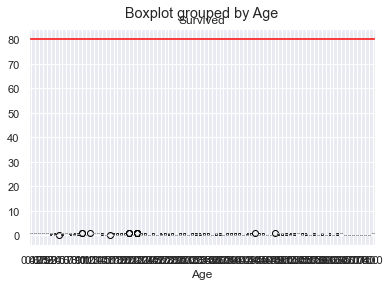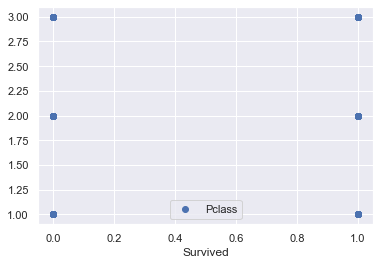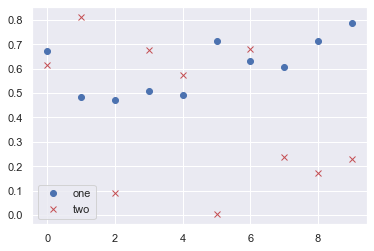## Project title: Titanic case study and Twitter sentiment analysis in machine learning (Hello world of ML)

In :
``# Question 1``
In :
``````import pandas as pd
``````
Out:

## Data Preparation and Cleaning

The training-set has 891 examples and 11 features + the target variable (survived). 2 of the features are floats, 5 are integers and 5 are objects.

In :
``d.drop(["Name","PassengerId","Parch","Ticket","Cabin","Embarked"], axis=1, inplace= True)``
In :
``d.head()``
Out:
In :
``````d.loc[d['Sex'] =='male', 'Sex'] = 1
d.loc[d['Sex'] =='female', 'Sex']= 0``````
In :
``d.head()``
Out:

Age: Now we can tackle the issue with the age features missing values. I will create an array that contains random numbers, which are computed based on the mean age value in regards to the standard deviation and is_null.

In :
``````d['Age'][(d['Pclass'] == 1) & (d['Age'].isnull())] = 37
d['Age'][(d['Pclass'] == 2) & (d['Age'].isnull())] = 30
d['Age'][(d['Pclass'] == 3) & (d['Age'].isnull())] = 24

``````
```C:\Users\Garima Singh\Anaconda3\lib\site-packages\ipykernel_launcher.py:1: SettingWithCopyWarning: A value is trying to be set on a copy of a slice from a DataFrame See the caveats in the documentation: https://pandas.pydata.org/pandas-docs/stable/user_guide/indexing.html#returning-a-view-versus-a-copy """Entry point for launching an IPython kernel. C:\Users\Garima Singh\Anaconda3\lib\site-packages\ipykernel_launcher.py:2: SettingWithCopyWarning: A value is trying to be set on a copy of a slice from a DataFrame See the caveats in the documentation: https://pandas.pydata.org/pandas-docs/stable/user_guide/indexing.html#returning-a-view-versus-a-copy C:\Users\Garima Singh\Anaconda3\lib\site-packages\ipykernel_launcher.py:3: SettingWithCopyWarning: A value is trying to be set on a copy of a slice from a DataFrame See the caveats in the documentation: https://pandas.pydata.org/pandas-docs/stable/user_guide/indexing.html#returning-a-view-versus-a-copy This is separate from the ipykernel package so we can avoid doing imports until ```
In :
``d.head()``
Out:
In :
``d``
Out:

From the table above, we can note a few things. First of all, that we need to convert a lot of features into numeric ones later on, so that the machine learning algorithms can process them. Furthermore, we can see that the features have widely different ranges, that we will need to convert into roughly the same scale. We can also spot some more features, that contain missing values (NaN = not a number), that wee need to deal with.

In :
``````d.fillna(d.mean())
d
``````
Out:

## Exploratory Analysis and Visualization

The Embarked feature has only 2 missing values, which can easily be filled. It will be much more tricky, to deal with the ‘Age’ feature, which has 177 missing values. The ‘Cabin’ feature needs further investigation, but it looks like that we might want to drop it from the dataset, since 77 % of it are missing.

In :
``````
X=d[['Pclass','Sex','Age','SibSp','Fare']] #dependent
y=d.Survived #independent

``````

Creating Categories: We will now create categories within the following features: Age: Now we need to convert the ‘age’ feature. First we will convert it from float into integer. Then we will create the new ‘AgeGroup” variable, by categorizing every age into a group. Note that it is important to place attention on how you form these groups, since you don’t want for example that 80% of your data falls into group 1.

In :
``````from sklearn.model_selection import train_test_split
X_train,X_test,y_train,y_test=train_test_split(X,y,test_size=0.30,random_state=42)

``````
In :
``````from sklearn.linear_model import LogisticRegression
logreg = LogisticRegression()

logreg.fit(X_train,y_train)

y_pred=logreg.predict(X_test)

from sklearn import metrics
cnf_matrix = metrics.confusion_matrix(y_test, y_pred)
cnf_matrix

``````
Out:
``````array([[139,  18],
[ 29,  82]], dtype=int64)``````

Building Machine Learning Models Now we will train several Machine Learning models and compare their results. Note that because the dataset does not provide labels for their testing-set, we need to use the predictions on the training set to compare the algorithms with each other. Later on, we will use cross validation.

In :
``````print("Accuracy:",metrics.accuracy_score(y_test, y_pred))
print("Precision:",metrics.precision_score(y_test, y_pred))
print("Recall:",metrics.recall_score(y_test, y_pred))

``````
```Accuracy: 0.8246268656716418 Precision: 0.82 Recall: 0.7387387387387387 ```
In :
``````import matplotlib
import matplotlib.pyplot as plt
import matplotlib.gridspec as gridspec``````
In :
``````ax = d.boxplot(column='Survived',by='Age');
ax.axhline(80,color='red')``````
Out:
``<matplotlib.lines.Line2D at 0x1e12efc6388>``In :
``````d.plot(x='Survived', y='Pclass', style='o')

import numpy as np
d = {'one' : np.random.rand(10),
'two' : np.random.rand(10)}

d = pd.DataFrame(d)

d.plot(style=['o','rx'])``````
Out:
``<matplotlib.axes._subplots.AxesSubplot at 0x1e13520b408>``In :
``````import seaborn as sns
import pandas.util.testing as tm
sns.set()``````
In [ ]:
``````x=["Survived"]
y=["Age"]
plt.plot(x, y)
plt.legend('ABCDEF', ncol=2, loc='upper left');``````
In [ ]:
``````from pandas import read_csv
from seaborn import distplot
from seaborn import boxplot
from matplotlib import pyplot

boxplot(x=0, data=d)
# show plot
pyplot.show()
``````

## Inferences and Conclusion

Summary We started with the data exploration where we got a feeling for the dataset, checked about missing data and learned which features are important. During this process we used seaborn and matplotlib to do the visualizations. During the data preprocessing part, we computed missing values, converted features into numeric ones, grouped values into categories and created a few new features. Afterwards we started training 8 different machine learning models, picked one of them (random forest) and applied cross validation on it. Then we discussed how random forest works, took a look at the importance it assigns to the different features and tuned it’s performace through optimizing it’s hyperparameter values. Lastly, we looked at it’s confusion matrix and computed the models precision, recall and f-score.

In :
``````# Question 2
In :
``````df=pd.read_csv("2Q.csv")
df

``````
Out:
In :
``````df["text"]= df["text"].str.lower()
df
``````
Out:
In :
``````df['text'] = df['text'].str.replace(r'[^\w\s]+', '')
df
``````
Out:
In :
``````import nltk
from nltk.corpus import stopwords``````
In :
``nltk.download('stopwords')``
```[nltk_data] Downloading package stopwords to C:\Users\Garima [nltk_data] Singh\AppData\Roaming\nltk_data... [nltk_data] Package stopwords is already up-to-date! ```
Out:
``True``
In :
``````stop = stopwords.words('english')

df['text'] = df['text'].apply(lambda x: ' '.join([word for word in x.split() if word not
in (stop)]))

df

``````
Out:
In :
``````from nltk.stem import PorterStemmer
from nltk.stem import LancasterStemmer
from nltk.stem.porter import PorterStemmer

def get_stemmed_text(corpus):
stemmer = PorterStemmer()
return [' '.join([stemmer.stem(word) for word in review.split()]) for review in corpus]

df['text'] = get_stemmed_text(df['text'])

``````
In :
``df``
Out:
In :
``````
```[nltk_data] Downloading package wordnet to C:\Users\Garima [nltk_data] Singh\AppData\Roaming\nltk_data... [nltk_data] Package wordnet is already up-to-date! ```
Out:
``True``
In :
``````from nltk.stem import WordNetLemmatizer

def get_lemmatized_text(corpus):
lemmatizer = WordNetLemmatizer()
return [' '.join([lemmatizer.lemmatize(word) for word in review.split()]) for review in corpus]

df['text'] = get_lemmatized_text(df['text'])``````
In :
``df``
Out:
In :
``df.to_csv('Pre-processed_text.csv')``
In [ ]:
`` ``
In [ ]:
`` ``
In [ ]:
`` ``
In [ ]:
`` ``
In [ ]:
`` ``